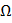# Three equal resistances, each ofohm, are connected as shown in figure. A battery of 2 V and internal resistance 0.1is connected across the circuit. The value offor which the heat generated in the circuit will by maximum isa) 0.3b) 0.01c) 0.1d) 0.03## Question ID - 151225 :- Three equal resistances, each ofohm, are connected as shown in figure. A battery of 2 V and internal resistance 0.1is connected across the circuit. The value offor which the heat generated in the circuit will by maximum isa) 0.3b) 0.01c) 0.1d) 0.033537

 (a) Total external resistanceFor maximum heat generationNext Question :

A truth table is given below. Which of the following has this type of truth table

 A 0 1 0 1 B 0 0 1 1 y 1 0 0 0

a)

XOR gate

b)

NOR gate

c)

AND gate

d)

OR gate